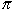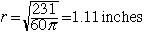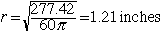I am trying to calculate the dia. of a pipe 60 inches long that will hold a gallon of water. What is the formula? Landry Hi Landry, First you need to know which gallon. A US gallon is 231 cubic inches and a British imperial gallon is 277.42 cubic inches. The volume of a cylinder isr 2 L where r is the radius and L is the length. Your cylinder is 60 inches long so 60r 2 = 231 or 60r 2 = 277.42 Henceif the gallons are US gallons orif the gallons are British imperial gallons. The diameter is twice the radius. Cheers, Penny Go to Math Central